## THE AMAZING NUMERICAL SYMMETRY MIRACLE IN THE QUR’AN – NEVER A HUMAN PRODUCT

God has created almost everything in this universe symmetrically. This seems to be the reflection of the verse in the design that we created everything with its “wife”. Interestingly, even the face of the man is created as two symmetrical pairs, almost all the basic organs are in pairs and their symmetrical structures.

Many of the planets, stars, even galaxies, molecules, human and animal bodies look significantly symmetrical.

If he created symmetrically the elements that make up the creative universe, it is expected that his holy book named Koran, which is known to be, was built with symmetrical features.

The Quran has numerical symmetry features from hundreds of different angles. This is such a symmetry; It seems impossible to discover a symmetry in this way even with the help of a computer. The number of 114 verses of the 114 suras chosen to create the Qur’an has been chosen so spectacularly that it shows extraordinary situations in many different respects. Let’s examine a few of these hundreds of symmetries together.

The table of surahs and verses in the Qur’an, which is accepted as common all over the world. We will use the table below in all our transactions.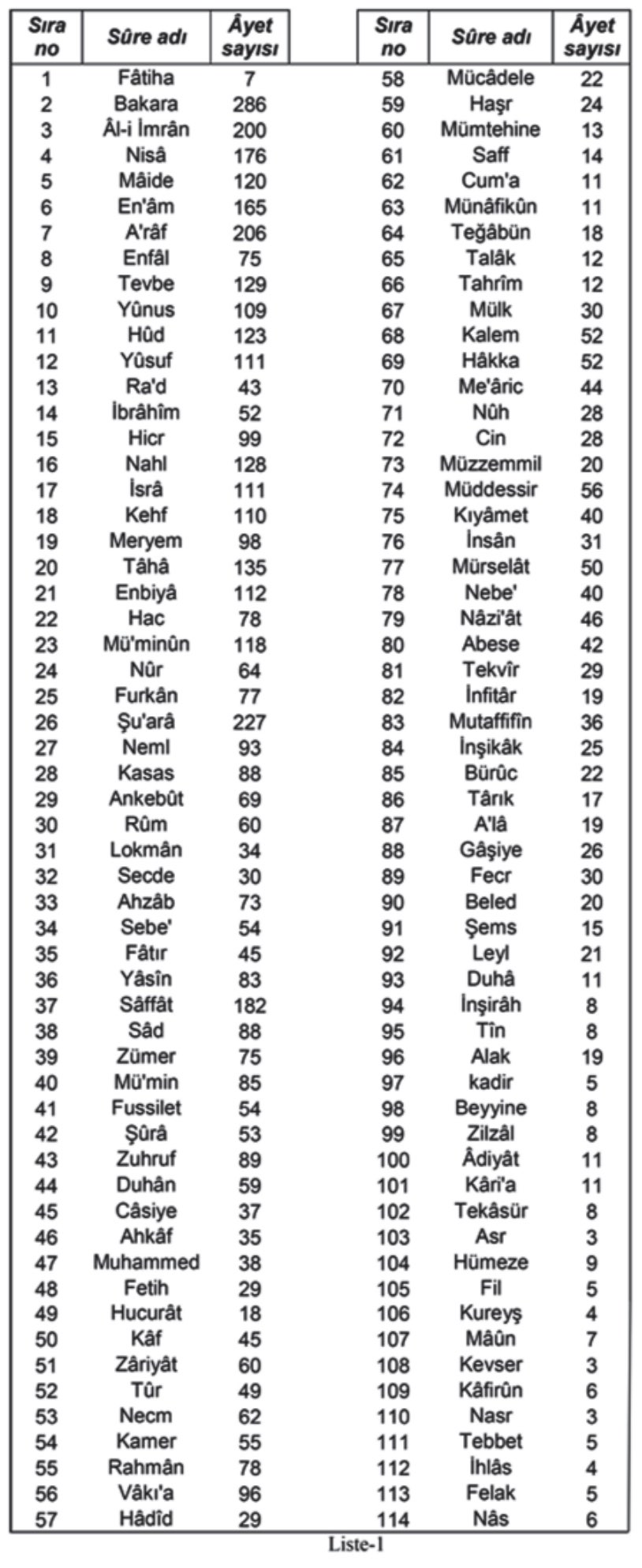## SYMMETRY IN QURAN IN SINGLE AND DOUBLE NUMBERS

The sequence number and verse numbers of suras in the Qur’an can be divided into two groups as single and double. Let’s explain the suras in order.

1 Surah Fatiha;

Sequence no 1 / Number of Verses 7

Odd number / Odd Number

In short, what we want to emphasize here is that the Sura of Fatiha will be located in the Single x Single part of the symmetry table.

2 Surat al-Baqara;

Order no 2 / Number of Verses 286

Even number / Even number

Baccarat time must be placed in the Double-Double column in the symmetry table. Because the number of rows and the number of verses consist of even numbers.

3 Surah Ali-Imran;

Order no.3 / Number of Verses 200

Odd / Even Number

Surat Ali İmran will be placed in the odd x even column in the symmetry table. Because the number of rows is single, the number of verses is even.

Thus, we list all the possibilities in the table, which we divide into 4 groups as single-single, double-double, single-double, double-single.

When we move each sura to the appropriate place in the table, we encounter a symmetrical cluster. You can see the list of this table, which is just one of the hundreds of symmetrical configurations and the simplest, by testing below.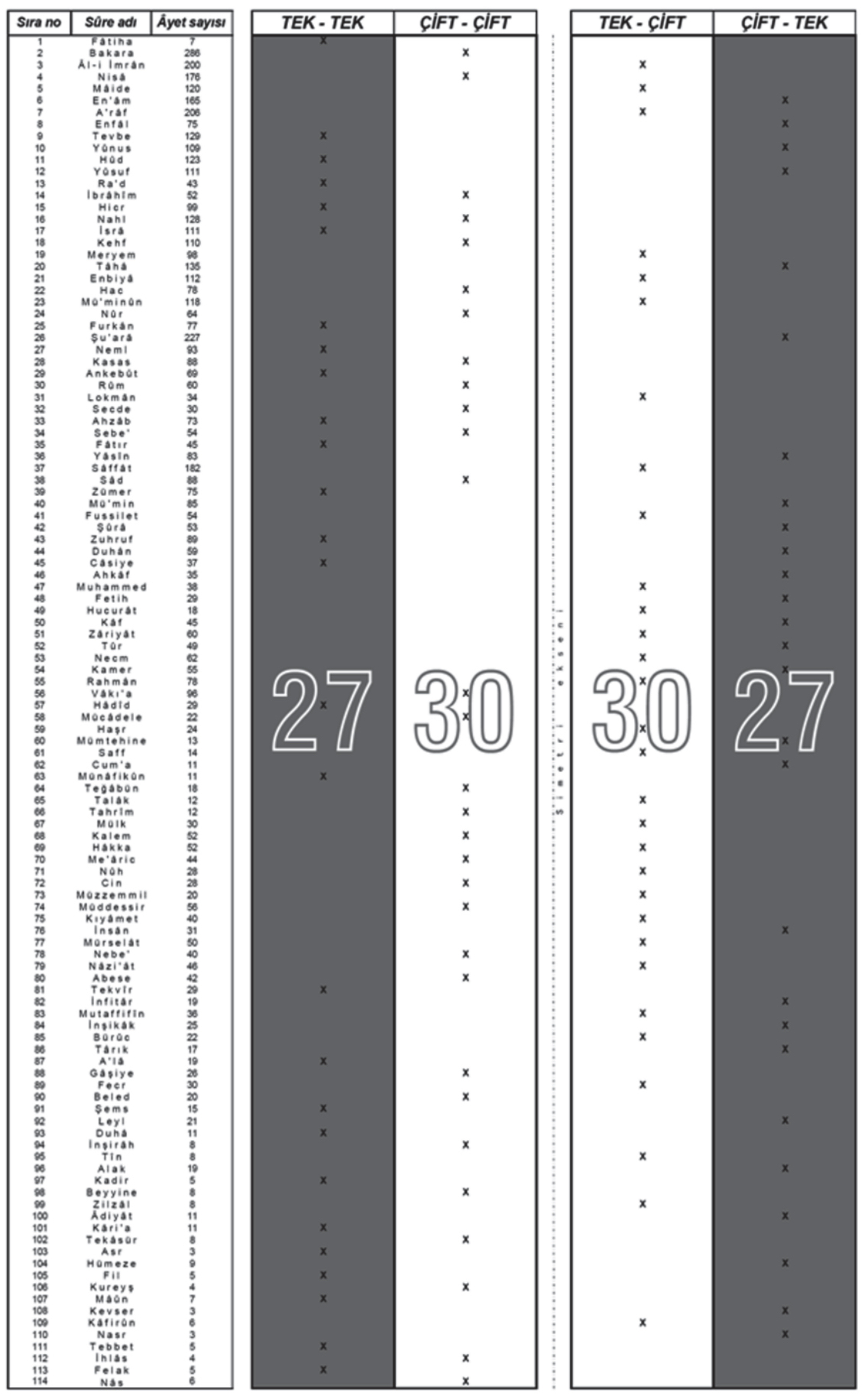THIS SYMMETRIC TABLE EXPOSES IN THE QURAN WHEN THE NUMBER OF SEQUENCES AND THE NUMBER OF VERSES IS EXAMINED;

27 Odd x Odd Number Sura = 27 Odd x Even Number Sura

30 Even x Even Number Sura = 30 Even x Even Number Sura

Let’s take this miracle one step further, let’s divide the Qur’an right from the middle, that is, from the 54th Sura, let’s take its symmetry. Here is the table below. Let’s group the suras as “homogeneous” and “non-homogeneous”. In other words, a period that has numerical values such as the time of Fatiha (Tek X Tek) should be written in the congener column. Likewise, the Surah Baccarat, which is a time with even X even numerical values, will be written in the Turks table. In this case;

THE FIRST HALF OF THE QUR’AN: 28 TÜRDEŞ NO SURE – 29 NON-TÜRDEŞ SURE

IN THE SECOND HALF OF THE QUR’AN: 29 SURE NUMBERED SURE – 28 NON-SMALL SURE

In other words, we encounter a symmetrical table both up and down and right-left.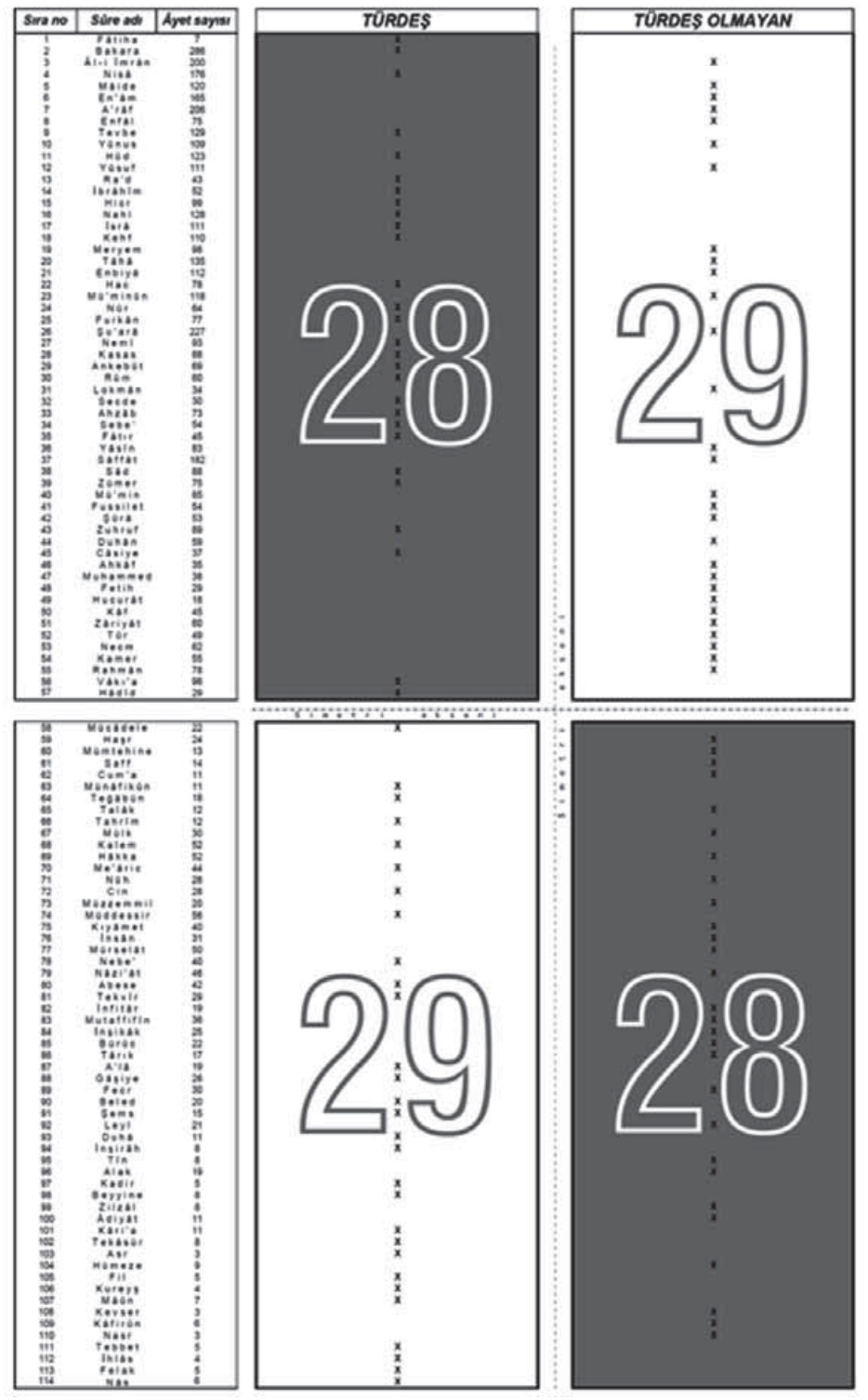## THE MIRACLE IN THE TOTAL NUMBER OF VERSES IN THE QUR’AN

There are 114 suras in the Qur’an. The sum of the sequence numbers of these suras is 6555. You can easily verify this information by calculating the sum of the numbers from 1 to 114 in order. ( 6555 )

The sum of the surahs in which the total number of queue numbers and verses in the Qur’an is TEK is 6555.

The total number of verses in 114 different suras, that is, the total number of verses in the Qur’an is 6236.

The sum of the surahs in the Qur’an, whose total number of queues and verses is DOUBLE, is 6236.

In other words, if we evaluate the suras as single and double in terms of the sum of numerical values; We access the total number of verses and sum of sura sequence numbers.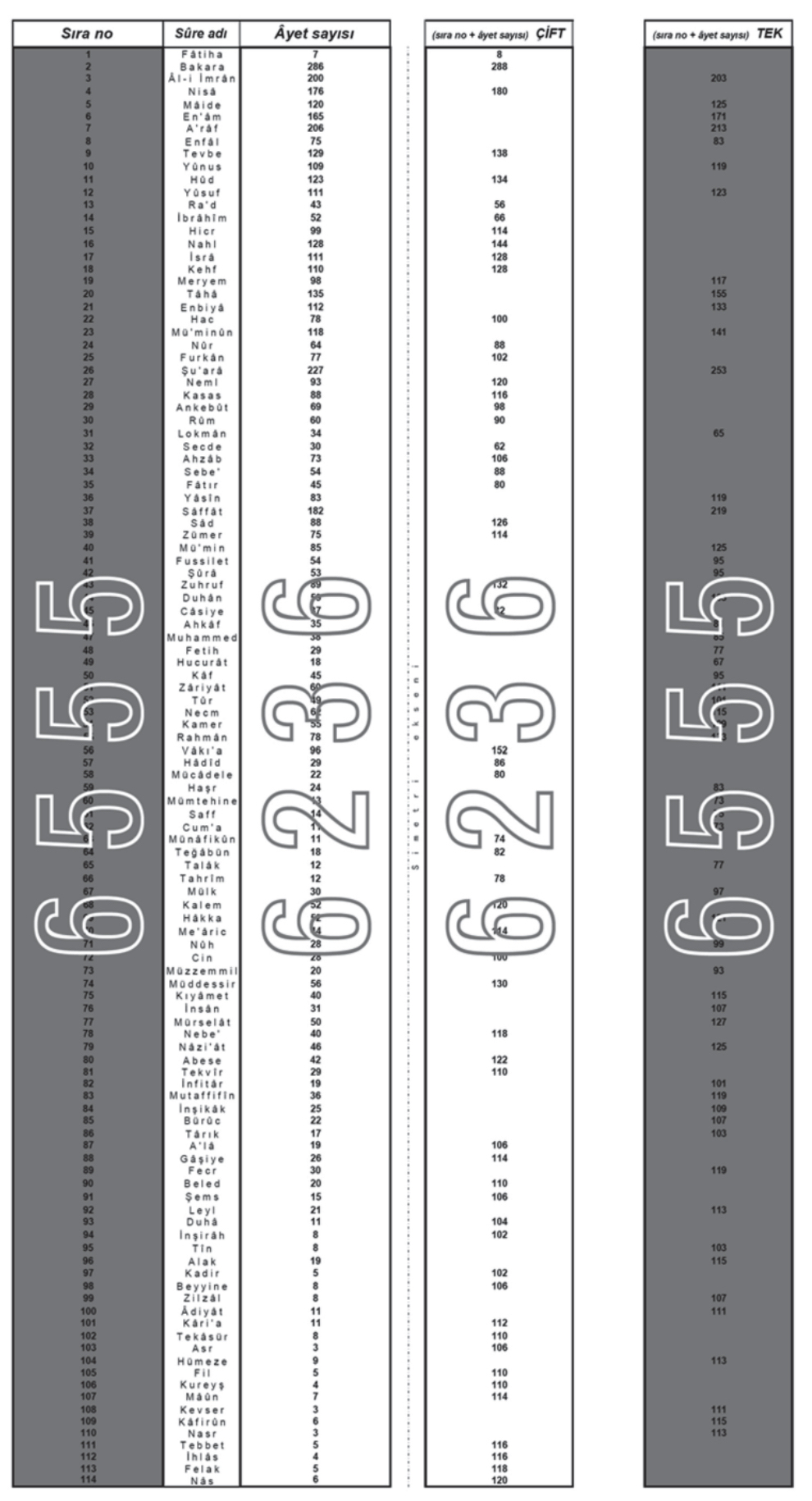Even when we group the Qur’anic suras as surahs larger than the number of verses in terms of single and double values, this balance does not deteriorate. In the tables below, you will see many symmetrical tables that have been studied from different angles.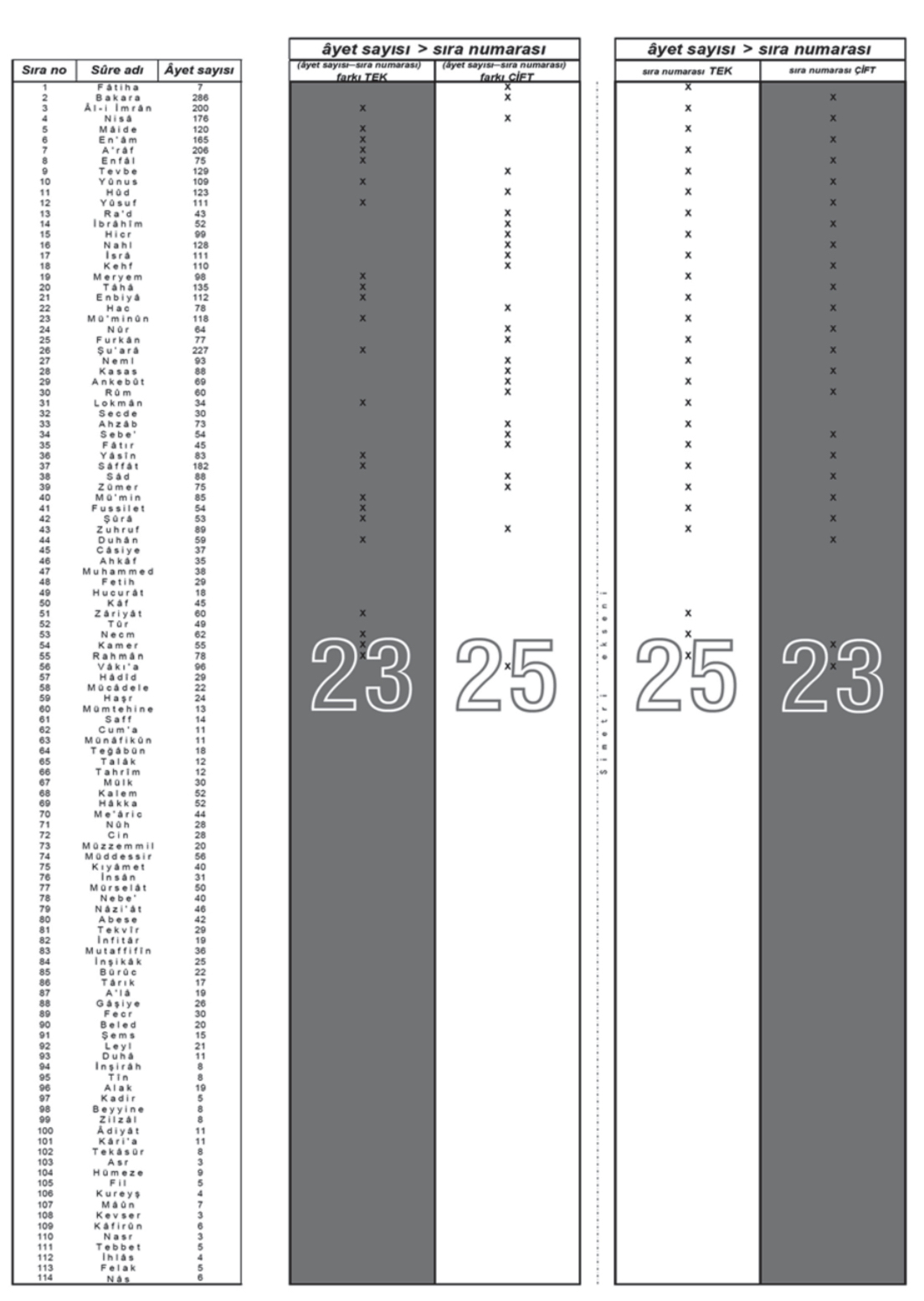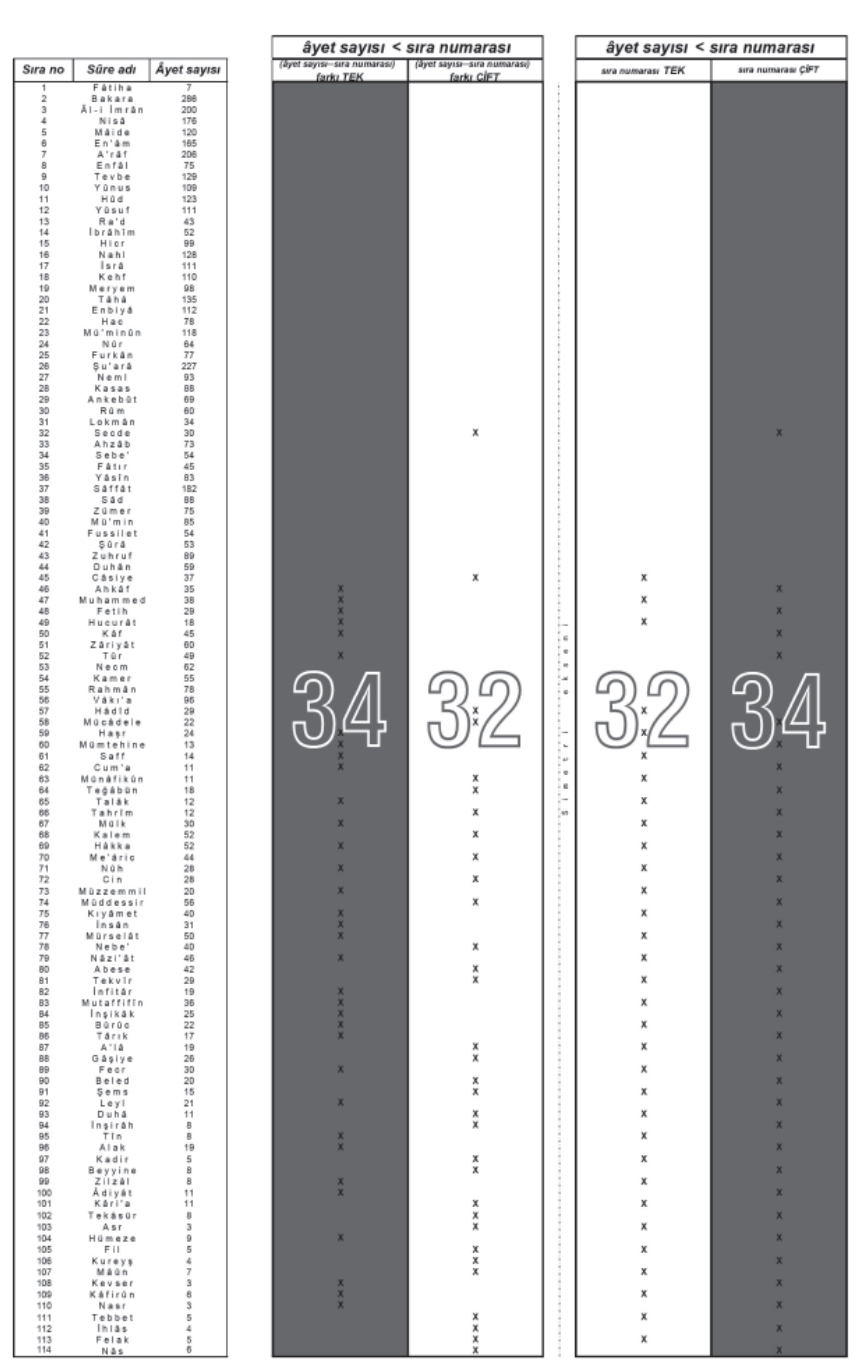## OTHER SYMMETRY MIRACLES

The symmetrical design in the Qur’an does not end with these. Symmetry can also be found in the figures below.

Prime numbers

Relationships Between Sets of Sequence Numbers and Sets of Verse Numbers

Numbers Divided Into Two But Not Divided By Numbers Divided Into Three But Not Divided By Prime Multipliers

Perfect Numbers

Rich Numbers

Scarce Numbers

Verse Numbers Arithmetic Average

Long Times and Short Times

Relationship Between Verse Numbers Arithmetic Averages

The location of the number 6236 ‘

Two Primes in the Division Set

Three Primes in the Division Set

Sequence Numbers and Verse Numbers of Surahs in Groups of 19… and more

If you want to prove all the explanations about the subject and examine the tables, please refer to Halis Aydemir, the 5th book of the series of Sacred Mysteries Publications, “Kerim Kerim; Read the book titled “Symmetrical Book”. Just email me to access the book.

### RELATED POSTS

#### 1 Comment

1.Selamlar
Çalışmalarınızı tebrik ederim. Allah kolaylık versin. Çok farklı nümerik mucizeler var ve siz bir çok yeni mucize bulmuşsunuz. Kitabınızı okumak isterim.